# How can the speed of light be constant for all observers?

• B
Karagoz
A rocket is in constant velocity. The velocity of the rocket is 150Mm/s (or 0.5 of the speed light, or 150 million meters per second) relative to us (we as observer).

We observe two lights, one moving in parallell with the rocket, another is moving in the opposite direction.

Below I have made a picture of what we (or observers staying still relative to the rocket outside) see in one moment (above), and what we see after one second (below). It's from our perspective.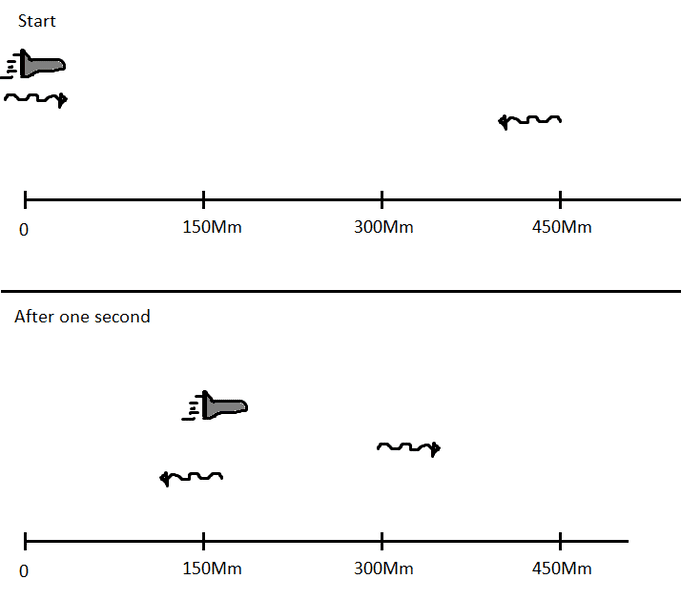The speed of light is constant for all observers. So the astronauts in the rocket will observe something different than us.

The Lorentz factor γ will be: 1/√(1-0.5^2) = 1.1547.

Their time be slower, so their time will be 1 = 1/γ = 1/1.1547 = 0.866.

So they'll experience our 1 second as 0.866 seconds.

And that means with the velocity 150 Mm/sec, they'll experience that they have traveled
0.866*150Mm = 129.9Mm.

The light has traveled 300Mm.

So with that calculation, they're 300-129.9 = 170.1Mm behind the light.

But since they too will observe the light to have same velocity, within 0.866 seconds they should see the light traveled past them by 259.8Mm (0.866*300).

And they should see the other light not reached them after 0.866 seconds or after one second.

How would the astronauts in the rocket observe what happened from that moment to one second after that moment illustrated in the pictured above?

#### Attachments

Last edited:

Google for "relativity of simultaneity", then use the full Lorentz transform rather than the time dilation formula, which is a special case that doesn't apply here.

Mentor
You better specify this a lot more carefully eg what frame is the rocket moving at .5 speed of light relative to. As it stands it beats me what you are getting at. In relativity you must be precise, very precise to the point its painful. When you do that it usually isn't that hard. But as Ibix said simultaneity is not preserved in relativity and you need the Full Lorentz Transformations.

Thanks
Bill

Mentz114
[..]

And they should see the other light not reached them after 0.866 seconds or after one second.

How would the astronauts in the rocket observe what happened from that moment to one second after that moment illustrated in the pictured above?
Here is a space-time diagram of your scenario. The blue line is the space ship and the dots are ticks on the space ship clock. The yellow lines are the light beams. Any horizontal line is a 'now' line for us, and where the now line intercepts the world lines of of the ship and light gives their positions at that time.

It will help you to know that the speed of light in these diagrams is 1 which is why the slope of the light lines is 1. This means that the X units are the distance tavelled by light in whatever unit is assigned to the time axis. If we assign 1 second per time tick, the X unit is light-second = c x 1 sec. You can choose any unit for the time axis that suits you.

Notice that in our frame the red now will be 11 ticks on our clock but about 9.6 ticks on the ship clock.

I've also posted a (crude) animation here.

http://www.blatword.co.uk/space-time/minkowski-anim-1.avi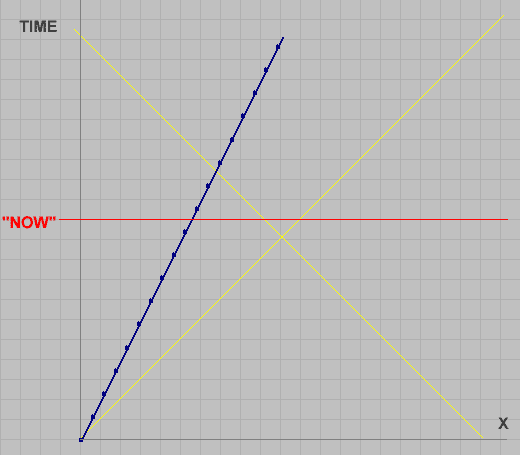#### Attachments

Last edited:
•vanhees71
Karagoz
Here is a space-time diagram of your scenario. (. . .)

Do your diagram reflect how is it observed by us?

From what I understand is this:
We observe both light waves start moving in the same moment.

But the astronauts in the rocket will see the light waves start moving in different times.

But which one will start moving first for the astronauts?

Mentz114
Do your diagram reflect how is it observed by us?
Yes. The rocket is moving at c/2 wrt us.
From what I understand is this:
We observe both light waves start moving in the same moment.
Yes, the lights start at the same time on our clocks.

But the astronauts in the rocket will see the light waves start moving in different times.
Yes, they see the distant light start much earlier ( that is - on their clock the distant light started much earlier than they did)
But which one will start moving first for the astronauts?
The light at their starting location starts at the same time as the ship on all clocks because they are co-located in space and time.

This is the diagram if we match speed with the ship. This is a different diagram from the earlier one. The number of ticks between two events on a worldline is invariant under Lorentz transformation as is the speed of light.
In our coordinates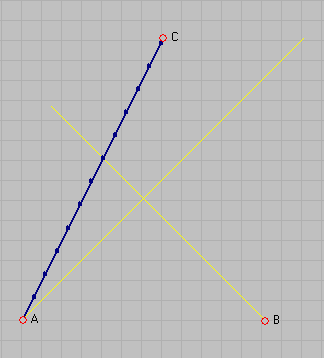In the ship coordinatess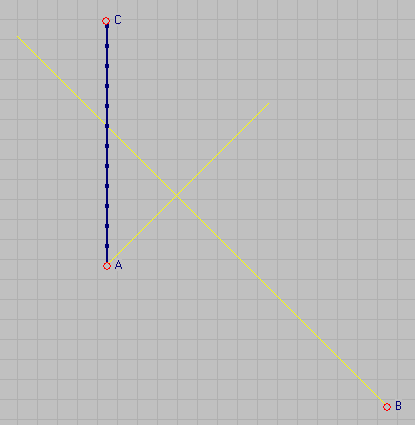#### Attachments

Last edited:
•vanhees71 and Karagoz
Karagoz
(. . .) Yes, they see the distant light start much earlier ( that is - on their clock the distant light started much earlier than they did)
(. . .)

Distance between the rocket and the light source; is it observed differently for us and for astrounts?

A similar picture here. It's similar to the "train example" on relativity of simultaneity.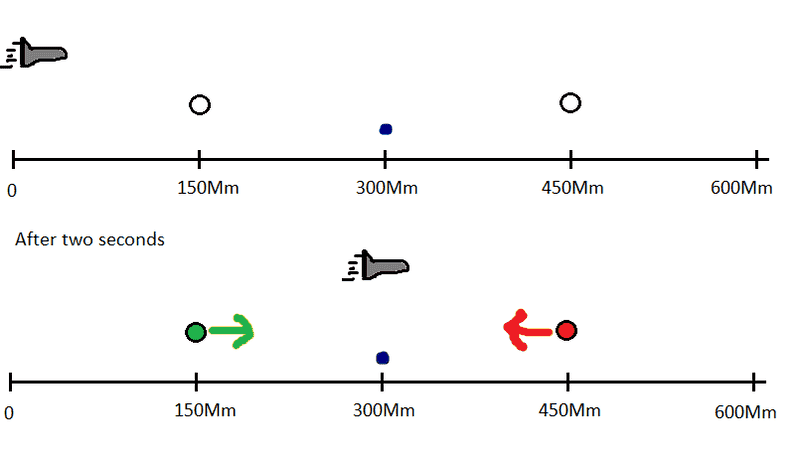We are placed on where the dark-blue dot is in the picture. The speed of rocket relative to us is 150 Mm/sec (0.5 c).

After two seconds when the rocket is in between two big lamps, the lamps send light waves simultaneously, red and green light.

Here's a schematic picture from our coordinates: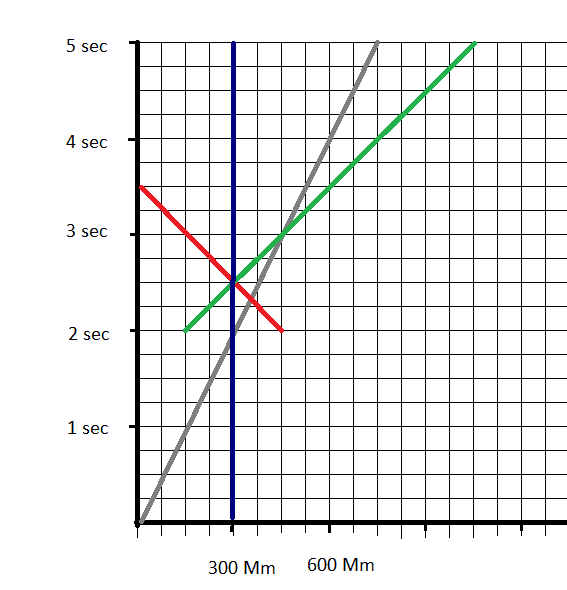We see that the lamps send light simultaneously, and both reach us at the same time. But we see that the red light reaches the rocket before the green light.

But the astronauts in the rocket won't observe that both lamps send light simultaneously. They'll observe that the red lamp send light first, then the green lamp send the red light later.

For astronauts, it's the universe, us (the blue dot) and the lamps that are moving with a velocity of -150Mm/sec while they're on rest.

And they'll observe the distance between the lamps to be less than 300 Mm.

We'll observe the source of both light coming as 150Mm away from us.

But how will astronauts see the distance between the rocket and the light sources?

But will the astronauts observe both source of light having equal distance from the blue dot?

I mean will the astronauts observe that the blue dot is placed in the center (in the middle) of the of light sources?

Or will astronauts observe their rocket in the middle of the light sources?

#### Attachments

Last edited:
•vanhees71
Mentz114
Distance between the rocket and the light source; is it observed differently for us and for astrounts?

A similar picture here. It's similar to the "train example" on relativity of simultaneity.

View attachment 223134

[..]

We see that the lamps send light simultaneously, and both reach us at the same time. But we see that the red light reaches the rocket before the green light.

But the astronauts in the rocket won't observe that both lamps send light simultaneously. They'll observe that the red lamp send light first, then the green lamp send the red light later.

Your space-time diagram shows the lights starting simultaneously in the ship coordinates. The lights beams and the ship all meet at 3 seconds after the start on the ship clocks. This meeting will happen in all frames.

You can read off all the answers to your questions from the diagram. In our frame the lights do not start simultaneously.

Karagoz
Your space-time diagram shows the lights starting simultaneously in the ship coordinates. The lights beams and the ship all meet at 3 seconds after the start on the ship clocks. This meeting will happen in all frames.

You can read off all the answers to your questions from the diagram. In our frame the lights do not start simultaneously.

I have draw the diagram in our coordinates. And in this diagram the lights don't reach the rocket at the same time.

So in the ship coordinates the red lamp sends the light first, then the green lampa sends the light after?

Mentz114
I have draw the diagram in our coordinates. And in this diagram the lights don't reach the rocket at the same time.

So in the ship coordinates the red lamp sends the light first, then the green lampa sends the light after?
In our coordinates the ship is moving so it cannot be a vertical line.
If the blue line in your diagram is the ship, then the coordinates are the ships. The lights start on the same horizontal line, ie the same time. That means they are simultaneous in those coordinates by definition.

Staff Emeritus
Homework Helper
Gold Member
2021 Award
In our coordinates the ship is moving so it cannot be a vertical line.
It isn't. It is the grey line. We are the blue vertical line. As colour coded by the illustrations.

Mentz114
It isn't. It is the grey line. We are the blue vertical line. As colour coded by the illustrations.
OK. But the OP switched from our previous convention.

Staff Emeritus
Homework Helper
Gold Member
2021 Award
OK. But the OP switched from our previous convention.
I think the OP never acknowledged that as a "convention" here. I strongly suspect the OP has taken those images from somewhere and therefore uses whatever convention was used there. I think it is pretty clear from context what is what in the OP's diagrams.

Mentz114
I have draw the diagram in our coordinates. And in this diagram the lights don't reach the rocket at the same time.

So in the ship coordinates the red lamp sends the light first, then the green lampa sends the light after?
I misunderstood your diagram. I did not realize that the ship worldline is the grey line. The answer to your question is in the second diagram in post#6. As I said, in the ship coords the red light is sent before the take-off time.

Let us say that our coordinates (t,x) of event B are (0, 12), which I can read from the first diagram in post#6. To find the values in the ship coordinates we use the Lorentz transformation (β=v/c)

t' = ϒt - ϒβx
x' = ϒx - ϒβt

You can check that this calculation will give the coords of event B on the second diagram. Note that in general t is not equal to t', and for two events ( say F,G) if tF = tG then t'F is not necessarily equal to t'G which expresses the relativity of simultaneity.

Last edited:
Karagoz
I misunderstood your diagram. I did not realize that the ship worldline is the grey line. The answer to your question is in the second diagram in post#6. As I said, in the ship coords the red light is sent before the take-off time.

Let us say that our coordinates (t,x) of event B are (0, 12), which I can read from the first diagram in post#6. To find the values in the ship coordinates we use the Lorentz transformation (β=v/c)

t' = ϒt - ϒβx
x' = ϒx - ϒβt

You can check that this calculation will give the coords of event B on the second diagram. Note that in general t is not equal to t', and for two events ( say F,G) if tF = tG then t'F is not necessarily equal to t'G which expresses the relativity of simultaneity.

Sorry for not clarifying things. In the post #7 the diagram and picture shows another case and event than what the picture in post #1 shows. They picture the event from our coordinates. The grey line is the rocket (which is moving), the blue straight line is the blue dot (where we are observing the event). We are at rest in our coordinates, so the blue line is straight.
[I have drawn these images myself using Paint, this is why they're so amateurish and not exact].

From the formula:
t' = ϒt - ϒβx
x' = ϒx - ϒβt

If we express the coordinates as (x,t).

So in our coordinates, the red lamps sends the light at (1.5, 2) and green lamp sends the light at (0.5, 2).

The Lorentz factor here is: ϒ = 1/√(1-0.5^2) = 1.1547.

β = v/c = 150,000 / 300,000 = 0.5

Starting point of the red light in astronaut's coordinates:
t' = ϒt - ϒβx = 1.1547*2 - 1.1547*0.5*1.5 = 1.443375
x' = ϒx - ϒβt = 1.1547*1.5 - 1.1547*0.5*2 = 0.57735

Starting point of the green light in astronaut's coordinates:
t' = ϒt - ϒβx = 1.1547*2 - 1.1547*0.5*0.5 = 2.020725
x' = ϒx - ϒβt = 1.1547*0.5 - 1.1547*0.5*2 = -0.57735

In our coordinates:
The red light starts from (1.5, 2) (1.5 light seconds from the origo, and after 2 seconds).
The green light starts from (0.5, 2).

But in rocket's coordinates:
The red light starts from (0.5773, 1.4433)
The green light starts from (-0.5773, 2.0207)

In our coordinates the lights hit us at (1, 2.5). In the rocket's coordinates the lights hit us at:
t' = ϒt - ϒβx = 1.1547*2.5 - 1.1547*0.5*1 = 2.3094
x' = ϒx - ϒβt = 1.1547*1 - 1.1547*0.5*2.5 = -0.2887

In our coordinates we stay at (1, 0) when t = 0 in our time. In rocket's coordinates we stay at:

x' = ϒx - ϒβt = 1.1547*1 - 1.1547*0.5*0 = 1.1547.
Then the time in rocket's coordinates are:
t' = ϒt - ϒβx = 1.1547*0 - 1.1547*0.5*1 = -0.5773

Is the calculations correct?

Here's the rocket's coordinates. The grey straight line is the rocket, since it's the rocket's coordinates the rocket is at rest relative to itself (I think one is always at rest relative to itself right?), but we (represented in blue line) are moving relative to the rocket.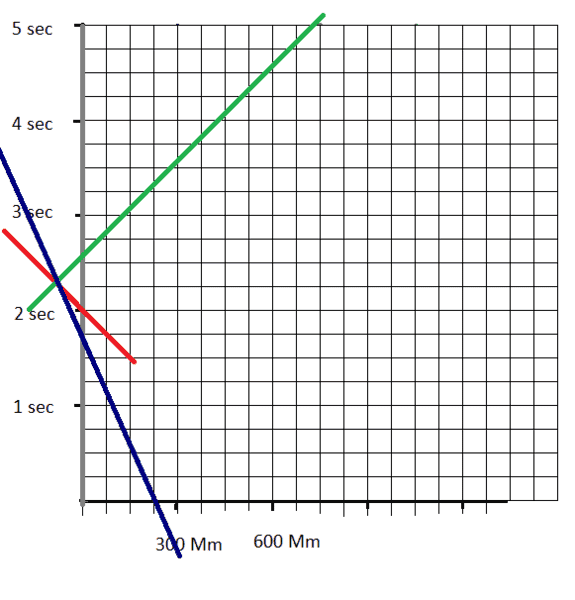Is this drawing correct?

#### Attachments

•Mentz114
Mentz114
Sorry for not clarifying things. In the post #7 the diagram and picture shows another case and event than what the picture in post #1 shows. They picture the event from our coordinates. The grey line is the rocket (which is moving), the blue straight line is the blue dot (where we are observing the event). We are at rest in our coordinates, so the blue line is straight.
[.]

Is the calculations correct?

Here's the rocket's coordinates. The grey straight line is the rocket, since it's the rocket's coordinates the rocket is at rest relative to itself (I think one is always at rest relative to itself right?), but we (represented in blue line) are moving relative to the rocket.

View attachment 223177

Is this drawing correct?
I don't have time to check each point but your diagram looks correct. It is similar to the second diagram in post#6. Your calculation shows that that the red light starts earlier than the green light in the space ship coordinates.

I hope you can see how the LT lets us calculate the coordinates of events for any inertial observer.
This transformation is passive because nothing changes - the coordinates are describing the same events.
The sort of questions you have asked can usually be answered by using the LT.

An interesting calculation is to transform ##t^2-x^2## to ##{t'}^2-{x'}^2## using the LT I gave. Do it algebraically and you'll get a surprise (maybe).

Last edited:
•Karagoz